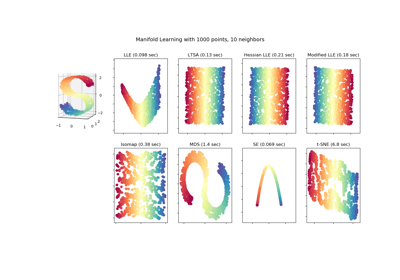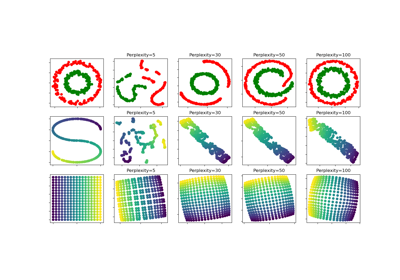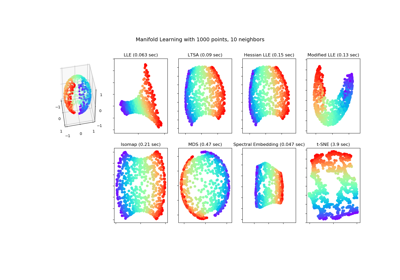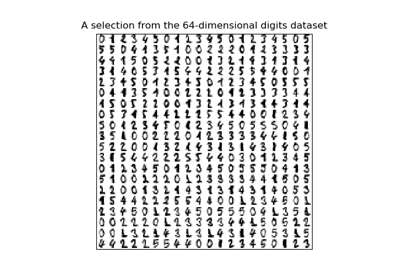# sklearn.manifold.TSNE¶

class sklearn.manifold.TSNE(n_components=2, perplexity=30.0, early_exaggeration=12.0, learning_rate=200.0, n_iter=1000, n_iter_without_progress=300, min_grad_norm=1e-07, metric='euclidean', init='random', verbose=0, random_state=None, method='barnes_hut', angle=0.5, n_jobs=None)[source]

t-distributed Stochastic Neighbor Embedding.

t-SNE  is a tool to visualize high-dimensional data. It converts similarities between data points to joint probabilities and tries to minimize the Kullback-Leibler divergence between the joint probabilities of the low-dimensional embedding and the high-dimensional data. t-SNE has a cost function that is not convex, i.e. with different initializations we can get different results.

It is highly recommended to use another dimensionality reduction method (e.g. PCA for dense data or TruncatedSVD for sparse data) to reduce the number of dimensions to a reasonable amount (e.g. 50) if the number of features is very high. This will suppress some noise and speed up the computation of pairwise distances between samples. For more tips see Laurens van der Maaten’s FAQ .

Read more in the User Guide.

Parameters
n_componentsint, optional (default: 2)

Dimension of the embedded space.

perplexityfloat, optional (default: 30)

The perplexity is related to the number of nearest neighbors that is used in other manifold learning algorithms. Larger datasets usually require a larger perplexity. Consider selecting a value between 5 and 50. Different values can result in significanlty different results.

early_exaggerationfloat, optional (default: 12.0)

Controls how tight natural clusters in the original space are in the embedded space and how much space will be between them. For larger values, the space between natural clusters will be larger in the embedded space. Again, the choice of this parameter is not very critical. If the cost function increases during initial optimization, the early exaggeration factor or the learning rate might be too high.

learning_ratefloat, optional (default: 200.0)

The learning rate for t-SNE is usually in the range [10.0, 1000.0]. If the learning rate is too high, the data may look like a ‘ball’ with any point approximately equidistant from its nearest neighbours. If the learning rate is too low, most points may look compressed in a dense cloud with few outliers. If the cost function gets stuck in a bad local minimum increasing the learning rate may help.

n_iterint, optional (default: 1000)

Maximum number of iterations for the optimization. Should be at least 250.

n_iter_without_progressint, optional (default: 300)

Maximum number of iterations without progress before we abort the optimization, used after 250 initial iterations with early exaggeration. Note that progress is only checked every 50 iterations so this value is rounded to the next multiple of 50.

New in version 0.17: parameter n_iter_without_progress to control stopping criteria.

If the gradient norm is below this threshold, the optimization will be stopped.

metricstring or callable, optional

The metric to use when calculating distance between instances in a feature array. If metric is a string, it must be one of the options allowed by scipy.spatial.distance.pdist for its metric parameter, or a metric listed in pairwise.PAIRWISE_DISTANCE_FUNCTIONS. If metric is “precomputed”, X is assumed to be a distance matrix. Alternatively, if metric is a callable function, it is called on each pair of instances (rows) and the resulting value recorded. The callable should take two arrays from X as input and return a value indicating the distance between them. The default is “euclidean” which is interpreted as squared euclidean distance.

initstring or numpy array, optional (default: “random”)

Initialization of embedding. Possible options are ‘random’, ‘pca’, and a numpy array of shape (n_samples, n_components). PCA initialization cannot be used with precomputed distances and is usually more globally stable than random initialization.

verboseint, optional (default: 0)

Verbosity level.

random_stateint, RandomState instance or None, optional (default: None)

If int, random_state is the seed used by the random number generator; If RandomState instance, random_state is the random number generator; If None, the random number generator is the RandomState instance used by np.random. Note that different initializations might result in different local minima of the cost function.

methodstring (default: ‘barnes_hut’)

By default the gradient calculation algorithm uses Barnes-Hut approximation running in O(NlogN) time. method=’exact’ will run on the slower, but exact, algorithm in O(N^2) time. The exact algorithm should be used when nearest-neighbor errors need to be better than 3%. However, the exact method cannot scale to millions of examples.

New in version 0.17: Approximate optimization method via the Barnes-Hut.

anglefloat (default: 0.5)

Only used if method=’barnes_hut’ This is the trade-off between speed and accuracy for Barnes-Hut T-SNE. ‘angle’ is the angular size (referred to as theta in ) of a distant node as measured from a point. If this size is below ‘angle’ then it is used as a summary node of all points contained within it. This method is not very sensitive to changes in this parameter in the range of 0.2 - 0.8. Angle less than 0.2 has quickly increasing computation time and angle greater 0.8 has quickly increasing error.

n_jobsint or None, optional (default=None)

The number of parallel jobs to run for neighbors search. This parameter has no impact when metric="precomputed" or (metric="euclidean" and method="exact"). None means 1 unless in a joblib.parallel_backend context. -1 means using all processors. See Glossary for more details.

New in version 0.22.

Attributes
embedding_array-like, shape (n_samples, n_components)

Stores the embedding vectors.

kl_divergence_float

Kullback-Leibler divergence after optimization.

n_iter_int

Number of iterations run.

References

 van der Maaten, L.J.P.; Hinton, G.E. Visualizing High-Dimensional Data

Using t-SNE. Journal of Machine Learning Research 9:2579-2605, 2008.

 van der Maaten, L.J.P. t-Distributed Stochastic Neighbor Embedding

https://lvdmaaten.github.io/tsne/

 L.J.P. van der Maaten. Accelerating t-SNE using Tree-Based Algorithms.

Journal of Machine Learning Research 15(Oct):3221-3245, 2014. https://lvdmaaten.github.io/publications/papers/JMLR_2014.pdf

Examples

>>> import numpy as np
>>> from sklearn.manifold import TSNE
>>> X = np.array([[0, 0, 0], [0, 1, 1], [1, 0, 1], [1, 1, 1]])
>>> X_embedded = TSNE(n_components=2).fit_transform(X)
>>> X_embedded.shape
(4, 2)


Methods

 fit(self, X[, y]) Fit X into an embedded space. fit_transform(self, X[, y]) Fit X into an embedded space and return that transformed output. get_params(self[, deep]) Get parameters for this estimator. set_params(self, \*\*params) Set the parameters of this estimator.
__init__(self, n_components=2, perplexity=30.0, early_exaggeration=12.0, learning_rate=200.0, n_iter=1000, n_iter_without_progress=300, min_grad_norm=1e-07, metric='euclidean', init='random', verbose=0, random_state=None, method='barnes_hut', angle=0.5, n_jobs=None)[source]

Initialize self. See help(type(self)) for accurate signature.

fit(self, X, y=None)[source]

Fit X into an embedded space.

Parameters
Xarray, shape (n_samples, n_features) or (n_samples, n_samples)

If the metric is ‘precomputed’ X must be a square distance matrix. Otherwise it contains a sample per row. If the method is ‘exact’, X may be a sparse matrix of type ‘csr’, ‘csc’ or ‘coo’. If the method is ‘barnes_hut’ and the metric is ‘precomputed’, X may be a precomputed sparse graph.

yIgnored
fit_transform(self, X, y=None)[source]

Fit X into an embedded space and return that transformed output.

Parameters
Xarray, shape (n_samples, n_features) or (n_samples, n_samples)

If the metric is ‘precomputed’ X must be a square distance matrix. Otherwise it contains a sample per row. If the method is ‘exact’, X may be a sparse matrix of type ‘csr’, ‘csc’ or ‘coo’. If the method is ‘barnes_hut’ and the metric is ‘precomputed’, X may be a precomputed sparse graph.

yIgnored
Returns
X_newarray, shape (n_samples, n_components)

Embedding of the training data in low-dimensional space.

get_params(self, deep=True)[source]

Get parameters for this estimator.

Parameters
deepbool, default=True

If True, will return the parameters for this estimator and contained subobjects that are estimators.

Returns
paramsmapping of string to any

Parameter names mapped to their values.

set_params(self, **params)[source]

Set the parameters of this estimator.

The method works on simple estimators as well as on nested objects (such as pipelines). The latter have parameters of the form <component>__<parameter> so that it’s possible to update each component of a nested object.

Parameters
**paramsdict

Estimator parameters.

Returns
selfobject

Estimator instance.

## Examples using sklearn.manifold.TSNE¶